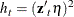# The AUTOREG Procedure

### HETERO Statement

• HETERO variables / options;

The HETERO statement specifies variables that are related to the heteroscedasticity of the residuals and the way these variables are used to model the error variance of the regression.

The heteroscedastic regression model supported by the HETERO statement is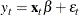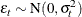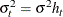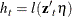The HETERO statement specifies a model for the conditional variance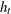. The vector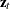is composed of the variables listed in the HETERO statement,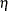is a parameter vector, and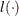is a link function that depends on the value of the LINK= option. In the printed output,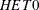represents the estimate of sigma, while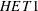-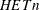are the estimates of parameters in thevector.

The keyword XBETA can be used in the variables list to refer to the model predicted value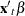. If XBETA is specified in the variables list, other variables in the HETERO statement will be ignored. In addition, XBETA cannot be specified in the GARCH process.

For heteroscedastic regression models without GARCH effects, the errors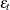are assumed to be uncorrelated — the heteroscedasticity models specified by the HETERO statement cannot be combined with an autoregressive model for the errors. Thus, when a HETERO statement is used, the NLAG= option cannot be specified unless the GARCH= option is also specified.

You can specify the following options in the HETERO statement.

specifies the functional form of the heteroscedasticity model. By default, LINK=EXP. If you specify a GARCH model with the HETERO statement, the model is estimated using LINK= LINEAR only. For details, see the section Using the HETERO Statement with GARCH Models. Values of the LINK= option are as follows:

EXP

specifies the exponential link function. The following model is estimated when you specify LINK=EXP: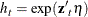SQUARE

specifies the square link function. The following model is estimated when you specify LINK=SQUARE: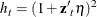LINEAR

specifies the linear function; that is, the HETERO statement variables predict the error variance linearly. The following model is estimated when you specify LINK=LINEAR: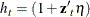COEF=value

imposes constraints on the estimated parametersof the heteroscedasticity model. You can specify the following values:

NONNEG

specifies that the estimated heteroscedasticity parametersmust be nonnegative.

UNIT

constrains all heteroscedasticity parametersto equal 1.

ZERO

constrains all heteroscedasticity parametersto equal 0.

UNREST

specifies unrestricted estimation of.

If you specify the GARCH= option in the MODEL statement, the default is COEF=NONNEG. If you do not specify the GARCH= option in the MODEL statement, the default is COEF=UNREST.

STD=value

imposes constraints on the estimated standard deviationof the heteroscedasticity model. You can specify the following values:

NONNEG

specifies that the estimated standard deviation parametermust be nonnegative.

UNIT

constrains the standard deviation parameterto equal 1.

UNREST

specifies unrestricted estimation of.

The default is STD=UNREST.

TEST=LM

produces a Lagrange multiplier test for heteroscedasticity. The null hypothesis is homoscedasticity; the alternative hypothesis is heteroscedasticity of the form specified by the HETERO statement. The power of the test depends on the variables specified in the HETERO statement.

The test may give different results depending on the functional form specified by the LINK= option. However, in many cases the test does not depend on the LINK= option. The test is invariant to the form ofwhen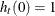and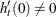. (The conditionis satisfied except when the NOCONST option is specified with LINK=SQUARE or LINK=LINEAR.)

NOCONST

specifies that the heteroscedasticity model does not include the unit term for the LINK=SQUARE and LINK=LINEAR options. For example, the following model is estimated when you specify the options LINK=SQUARE NOCONST: